TitleCollege Algebra
Answer/Discussion to Practice Problems
Tutorial 51: Systems of Linear Equations and Problem SolvingAnswer/Discussion to 1a The larger of two numbers is 5 more than twice the smaller.  If the smaller is subtracted from the larger, the result is 12.  Find the numbers.

 Make sure that you read the question carefully several times.  Since we are looking for two numbers, we will let    x = the smaller number  y = the larger number

 Step 2:  Devise a plan (translate).

 Since we have two unknowns, we need to build a system with two equations. Equation (1):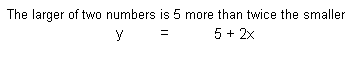Equation (2):Putting the two equations together in a system we get: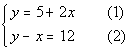This is a system of linear equations with two variables, which can be found in Tutorial 49: Solving Systems of Linear Equations in Two Variables. No simplification is needed for this problem.    I'm going to go ahead and have us use the substitution method as shown in Tutorial 49: Solving Systems of Linear Equations in Two Variables to solve this. Solve one equation for either variable Since equation (1) is nicely solved for y, we will use that to substitute into the other equation. y = 5 + 2x  (1)   Substitute what you get above into the other equation AND solve for the remaining variable. Substituting in 5 + 2x for y into equation (2) and solving for x we get: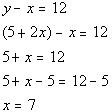*Substitute 5 + 2x in for y   *Inverse of add 5 is sub. 5

 Solve for second variable. Using equation (1) to plug in 7 for x and solving for y we get: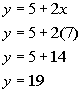*Equation (1) *Plug in 7 for x

 You will find that if you plug the ordered pair (7, 19) into BOTH equations of the original system, this is a solution to BOTH of  them.  Final Answer: The numbers are 7 and 19.Answer/Discussion to 1b It takes a boat 2 hours to travel 24 miles downstream and 3 hours to travel 18 miles upstream.  What is the speed of the boat in still water and of the current of the river?

Make sure that you read the question carefully several times.

Since we are looking for two different rates, we will let

x = rate of the boat

y = the rate of the current

Since this is a rate/distance problem, it might be good to organize our information using the distance formula.

Keep in mind that the rate of the current is affecting the overall speed.

When the boat is going upstream, it will be going slower.  That rate will be x - y

When the boat is going downstream, it will be going faster.  That rate will be x + y.

 (Rate) (Time) =  Distance Upstream x - y 3 18 Downstream x + y 2 24

 Step 2:  Devise a plan (translate).

 Since we have two unknowns, we need to build a system with two equations. Equation (1):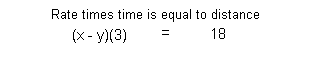Equation (2):Putting the two equations together in a system we get:This is a system of linear equations with two variables, which can be found in Tutorial 49: Solving Systems of Linear Equations in Two Variables. Simplify if needed.  We can simplify this by dividing both sides of equation (1) by 3 and equation (2) by 2:*Div. both side of eq. (1) by 3 *Div. both sides of eq. (2) by 2

 At this point, you can use any method that you want to solve this system.  I'm going to use the elimination method as discussed in Tutorial 49: Solving Systems of Linear Equations in Two Variables.   Multiply one or both equations by a number that will create opposite coefficients for either x or y if needed AND add the equations. Since we already have opposite coefficients on y, we can go right into adding equations (3) and (4) together: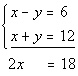*y's have opposite coefficients *y's dropped out

 Solve for remaining variable. Solving for x we get:*Inverse of div. by 2 is mult. by 2

 Solve for second variable. Using equation (4) to plug in 9 for x and solving for y we get: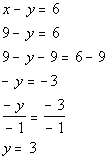*Equation (4) *Plug in 9 for x *Inverse of add 9 is sub. 9   *Inverse of mult. by -1 is div. by -1

 You will find that if you plug the ordered pair (9, 3) into BOTH equations of the original system, this is a solution to BOTH of  them.  Final Answer: The boat speed is 9 mph and the rate of the current is 3 mph.Answer/Discussion to 1c A student has money in three accounts that pay 5%, 7%, and 8%, in annual interest.  She has three times as much invested at 8% as she does at 5%.  If the total amount she has invested is \$1600 and her interest for the year comes to \$115, how much money does she have in each account?

 Make sure that you read the question carefully several times.  Since we are looking for three different amounts, we will let    x = the amount invested at 5% y = the amount invested at 7% z = the amount invested at 8%

 Step 2:  Devise a plan (translate).

 Since we have three unknowns, we need to build a system with three equations. Equation (1):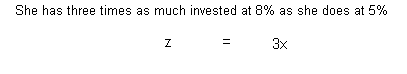Equation (2):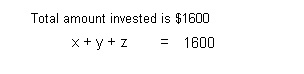Equation (3):Putting the three equations together in a system we get: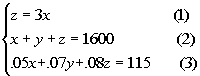This is a system of linear equations with three variables, which can be found in Tutorial 50:  Solving Systems of Linear Equations in Three Variables.   Simplify and put all three equations in the form Ax + By  + Cz = D if needed. Equation (1) needs to be put in the correct form and equation (3) needs to be multiplied by 100 to get rid of the decimals:*Inverse of add 3x is sub. 3x *Mult. both sides of eq. (3) by 100

 Choose to eliminate any one of the variables from any  pair of equations. Since y is already eliminated in equation (4), it would be quickest and easiest to eliminate y. We can use equation (4) as one equation with y eliminated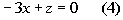*y is already eliminated

 Eliminate the SAME variable chosen in step 2 from any other pair of equations, creating a system of two equations and two unknowns.   Multiplying equation (2) by -7 and adding it to equation (5) we get: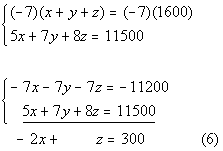*Mult. both sides of eq. (2) by -7       *y's have opposite coefficients *y's dropped out

 Solve the remaining system found above. Putting those two equations together we get:I'm going to choose to eliminate z. Multiplying equation (6) by -1 and then adding that to equation (4) we get: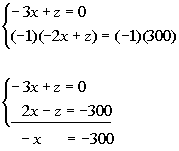*Mult. both sides of eq. (6) by -1     *z's have opposite coefficients *z's dropped out

 Solving for x we get: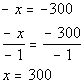*Inverse of mult. by -1 is div. by -1

 Using equation (4) to plug in 300 for x and solving for z we get:*Equation (4) *Plug in 300 for x   *Inverse of sub. 900 is add 900

 Solve for the third variable. Using equation (2) to plug in 300 for x and 900 for z we get: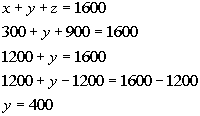*Equation (2) *Plug in 300 for x and 900 for z   *Inverse of add 1200 is sub. 1200

 You will find that if you plug the ordered triple (300, 400, 900) into ALL THREE equations of the original system, this is a solution to ALL THREE of  them.    Final Answer: \$300 is invested at 5%, \$400 is invested at 7% and \$900 is invested at 8%.

Last revised on April 25, 2011 by Kim Seward.
All contents copyright (C) 2002 - 2011, WTAMU and Kim Seward. All rights reserved.